# Solution assignment 03 Quadratic inequalities

### Assignment 3

Solve: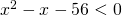### Solution

First we solve the corresponding equation: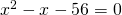which can be solved by factorizing: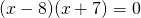and thus the solutions are: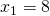or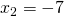The graph of the function in the left-hand side of the inequality is an 'opens up' parabola and thus the following values ofsatisfy the inequality: